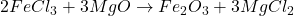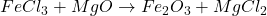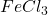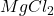## balance FeCl3 + MgO => Fe2O3 + MgCl2

Question

balance FeCl3 + MgO —> Fe2O3 + MgCl2

in progress 0
1 month 2021-08-15T05:00:09+00:00 1 Answers 2 views 0

1. Answer: The balanced equation isExplanation:

The given reaction equation is as follows.Here, number of atoms present on reactant side are as follows.

• Fe = 1
• Cl = 3
• Mg = 1
• O = 1

Number of atoms on product side are as follows.

• Fe = 2
• Cl = 2
• Mg = 1
• O = 3

To balance this equation, multiplyby 2 and MgO by 3 on reactant side. Also, multiplyby 3 on product side. Therefore, the equation can be rewritten as follows.Hence, number of atoms on reactant side are as follows.

• Fe = 2
• Cl = 6
• Mg = 3
• O = 3

Number of atoms on product side are as follows.

• Fe = 2
• Cl = 6
• Mg = 3
• O = 3

Since, this equation contains same number of atoms on both reactant and product side. Therefore, this equation is now balanced equation.

Thus, we can conclude that the balanced equation is.